# Before we start: Dataset

`import Algorithmiaimport pandas as pdclean_list=[]input={    'domains':'cnn.com',    'topic':'politics',    'q':'',    'qInTitle':'',    'content':'false',    'page':'1',    'author_only':'false'    }client = Algorithmia.client('ENTER_YOUR_ALGO_KEY')algo = client.algo('specrom/Historical_News_API/0.2.2')raw_dict = algo.pipe(input).resultclean_list = clean_list + raw_dict["Article"]news_df=pd.DataFrame(clean_list)news_df.head()`

# NLP

“Natural language processing (NLP) is the field of understanding human language using computers.”

# Explanatory Data Analysis

## Tokenization

`import pandas as pdimport numpy as npimport seaborn as snsimport matplotlib.pyplot as pltimport nltknltk.download()`
`title_list=news_df['title'].str.cat(sep='')from nltk.tokenize import word_tokenizetok_title= word_tokenize(title_list)`

## Stopword and Punctuation Removal

`corpus=[]corpus=[word for word in tok_title]from nltk.corpus import stopwordsstop=set(stopwords.words('english'))`
`new_stop_words=["'s","n't",'opinion','Video']for i in corpus:    if "CNN" in i:        new_stop_words.append(i)new_stop_words=list(set(new_stop_words))# adds the new stopwords to existing list of stop wordsstop=stop.union(new_stop_words)  `
`from string import punctuationcorpus=[char for char in corpus if (char not in punctuation)]corpus=[char for char in corpus if (char not in stop)]`
`from collections import Countercounter=Counter(corpus)most=counter.most_common()x,y=[],[]for word, count in most[:50]:    if word not in stop:        x.append(word)        y.append(count)        fig_dims=10,10fig,ax=plt.subplots(figsize=fig_dims)sns.barplot(x=y,y=x, ax=ax,palette=("Blues_d"))`

# NGram Exploration

`from sklearn.feature_extraction.text import CountVectorizerdef get_top_ngram(corpus, n=None):    vec = CountVectorizer(ngram_range=(n, n)).fit(corpus)    bag_of_words = vec.transform(corpus)    sum_words = bag_of_words.sum(axis=0)     words_freq = [(word, sum_words[0, idx])                   for word, idx in vec.vocabulary_.items()]    words_freq =sorted(words_freq, key = lambda x: x, reverse=True)    return words_freq[:10]top_n_bigrams=get_top_ngram(news_df['title'],2)[:10]x,y=map(list,zip(*top_n_bigrams))fig_dims=10,10fig,ax=plt.subplots(figsize=fig_dims)sns.barplot(x=y,y=x, ax=ax,palette=("Blues_r"))`
`top_n_trigrams=get_top_ngram(news_df['title'],3)[:10]x,y=map(list,zip(*top_n_trigrams))fig_dims=10,10fig,ax=plt.subplots(figsize=fig_dims)sns.barplot(x=y,y=x, ax=ax,palette=("Blues_r"))`
`top_n_pentagrams=get_top_ngram(news_df['title'],5)[:10]x,y=map(list,zip(*top_n_pentagrams))fig_dims=10,10fig,ax=plt.subplots(figsize=fig_dims)sns.barplot(x=y,y=x, ax=ax,palette=("Blues_r"))`

# Wordcloud

`from wordcloud import WordClouddef show_wordcloud(data):    wordcloud=WordCloud(background_color='white', stopwords=stop, max_words=100,                        max_font_size=30,scale=3,random_state=1)    wordcloud=wordcloud.generate(str(data))    fig = plt.figure(1, figsize=(15,15))    plt.axis('off')    plt.imshow(wordcloud)    plt.show()show_wordcloud(corpus)`

# Sentiment Analysis

`#Sentiment Analysisfrom textblob import TextBlobdef polarity(text):    return TextBlob(text).sentiment.polaritynews_df['polarity']=news_df['description'].apply(lambda x: polarity(x))news_df['polarity'].hist(figsize=(10,10))`
`['Early voting: Supreme Court moves in Pennsylvania and North Carolina set up potential post-election court fight over mail-in ballots - CNNPolitics',       'US election 2020: What India thinks of the US election (opinion) - CNN',"Where Trump and Biden stand in CNN's latest poll of polls","Biden says 'no excuse for looting' in wake of Wallace shooting - CNN Video",'Stay inside this Halloween with your household, doctors say - CNN']`

## Conclusion

--

--

--

Love podcasts or audiobooks? Learn on the go with our new app.

## Review — Vision Transformer with Deformable Attention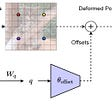## Getting Started with Discord ChatBot using Machine Learning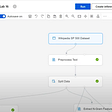## A simple introduction to Gradient Descent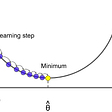## Intersection of Machine Learning and Health: Interview with Roberto Vega## Linear Regression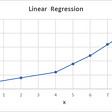## Word Embeddings : Intuition and (some) maths to understand end-to-end Skip-gram model## What is K-Means & GMMs — a dive into Expectation Maximization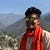## Achieving Sustainability Through Blockchain## Wait… is this just another “Restaurant Review Analysis” ?## With LOKO AI your own AI code follows you, wherever you need.